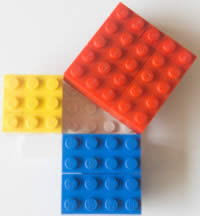# Pythagorean Triples

A "Pythagorean Triple" is a set of positive integers, a, b and c that fits the rule:

a2 + b2 = c2

### Example: The smallest Pythagorean Triple is 3, 4 and 5.

Let's check it:

32 + 42 = 52

Calculating this becomes:

9 + 16 = 25

Yes, it is a Pythagorean Triple!

## TrianglesWhen a triangle's sides are a Pythagorean Triple it is a right angled triangle.See Pythagoras' Theorem for more details.

### Example: The Pythagorean Triple of 3, 4 and 5 makes a Right Angled Triangle:Here are two more Pythagorean Triples:

 5, 12, 13 9, 40, 4152 + 122 = 132 92 + 402 = 412 25 + 144 = 169 (try it yourself)

And each triangle has a right angle!

## List of the First Few

Here is a list of the first few Pythagorean Triples (not including "scaled up" versions mentioned below):

 (3, 4, 5) (5, 12, 13) (7, 24, 25) (8, 15, 17) (9, 40, 41) (11, 60, 61) (12, 35, 37) (13, 84, 85) (15,112,113) (16, 63, 65) (17,144,145) (19,180,181) (20, 21, 29) (20, 99,101) (21,220,221) (23,264,265) (24,143,145) (25,312,313) (27,364,365) (28, 45, 53) (28,195,197) (29,420,421) (31,480,481) (32,255,257) (33, 56, 65) (33,544,545) (35,612,613) (36, 77, 85) (36,323,325) (37,684,685) ... infinitely many more ...

## Scale Them Up

The simplest way to create further Pythagorean Triples is to scale up a set of triples.

### Example: scale 3, 4, 5 by 2 gives 6, 8, 10

Which also fits the formula a2 + b2 = c2:

62 + 82 = 102

36 + 64 = 100